Courses
Courses for Kids
Free study material
Free LIVE classes
More

# Boxes and Sketches Class 5 Notes CBSE Maths Chapter 9 (Free PDF Download)LIVE
Join Vedantu’s FREE Mastercalss

## NCERT Class 5 Maths Chapter 9 Boxes and Sketches Revision Notes and Worksheets

NCERT Class 5 Maths syllabus introduces the concept of boxes and sketches in Chapter 9. You might have learned different types of 3D shapes in the previous classes. In this chapter, you will learn how boxes are made using sketches. Refer to the Boxes and Sketches revision notes to get a clear understanding of these concepts.

The revision notes are compiled following the CBSE guidelines and Class 5 standards. This simple explanation of these concepts will help you solve the exercises easily. Check out the worksheets and learn from the solutions prepared by our experts.

Last updated date: 25th Sep 2023
Total views: 144.3k
Views today: 3.44k

## 2D Shapes

• 2-dimensional shapes are flat with two dimensions: length and breadth.

• Squares, rectangles, circles, triangles, and other shapes are among them.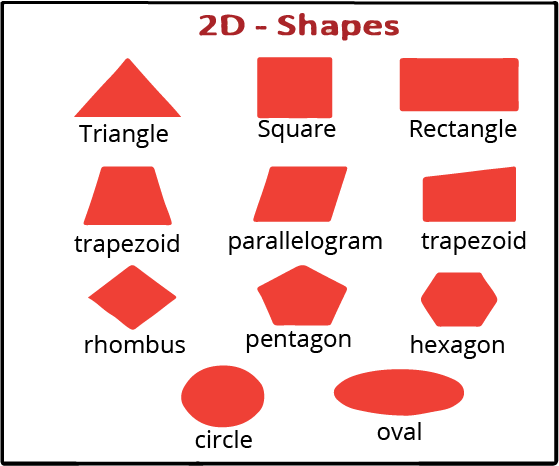2D Shapes

## 3D Shapes:

• A three-dimensional shape is described in geometry as a solid figure or an item or shape with three dimensions — length, breadth, and height.

• Three-dimensional forms, as opposed to two-dimensional shapes, have thickness or depth.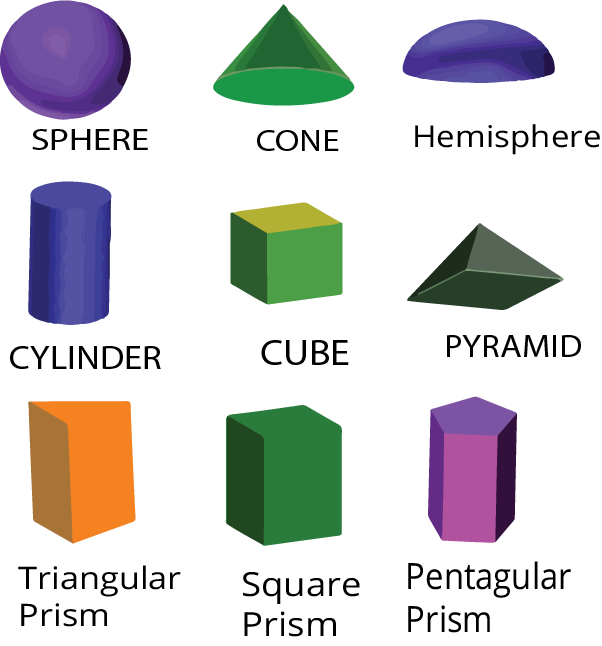3D Shapes

## Properties of 2D Shapes

### Circle

• A circle is a 2D closed-shape with no sides or edges made out of a curved line.

• A circle is made up of several different pieces, such as radius, diameter, and circumference.

• Coins, wheels, and pizzas are all instances of the circle in real life.

### Triangle

• A triangle is a closed shape that has three sides, three vertices, and three angles.

• It is a polygon with inner angles summing 180 degrees.

### Square

• A square is a two-dimensional form having four equal sides and each angle equal to 90 degrees.

• A loaf of bread and a chessboard are two real-life examples of squares.

### Rectangle

• A rectangle is a two-dimensional object having four sides that are equal and parallel, and all four angles measure 90 degrees.

• Tabletops, blackboards, cardboard, and other real-world instances of rectangles.

## Properties of 3D Shapes

### Sphere

• A sphere has only one curved surface.

• For example, planets, balls, globes, etc.

### Hemisphere

• A hemisphere has a single face, one curved surface, and one edge.

• For example, a scoop of ice cream, cups, etc.

### Cone

• A cone has a single face, one curved surface, one edge, and one vertex.

• For example, ice cream cones, traffic cones, etc.

### Cube

• A cube is a solid three-dimensional object. It has six faces, twelve edges, and eight vertices.

• For example, ice cubes, gift boxes, etc.

### Cylinder

• A cylinder is made up of two faces, one curved surface, and two edges.

• For example, battery, test tube, etc.

## Solved Example on 2D and 3D Shapes

1. Each cube and cuboid contains _____ plane surfaces,______ edges, and _____vertices.

Ans: six, twelve, eight

2. A ______ has neither a vertex nor an edge.

Ans: Sphere

## 2D to 3D shape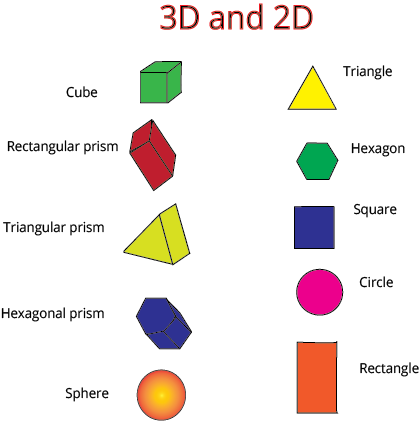2D Vs 3D Shapes

• Triangle is a 2D shape that can be converted into a pyramid or a cone when it is converted into a 3D shape.

• Circle is a 2D shape that can be converted into a sphere when it is converted into a 3D shape.

• Square is a 2D shape that can be converted into a cube when it is converted into a 3D shape.

• Rectangle is a 2D shape that can be converted into a cuboid when it is converted into a 3D shape.

## 2D representation of 3D objects

• Net is a 2D depiction of a 3D form. Flat, plane forms are 2D shapes.

• Three dimensions characterize 3D shapes: length, breadth, and depth.

• Architects create 2D drawings of 3D shapes, known as plans and elevations, to visualize how a structure will appear.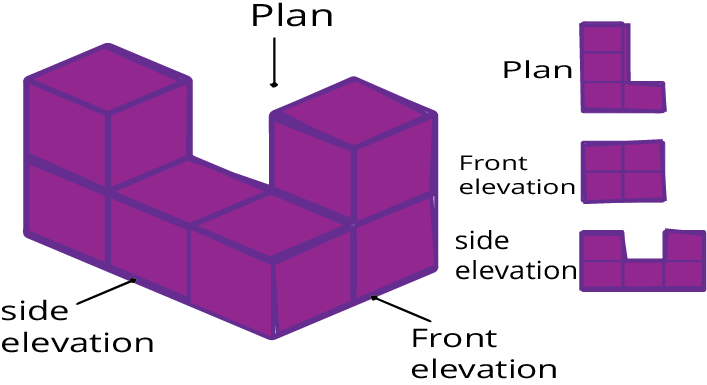2D representation of 3D objects

## Floor Maps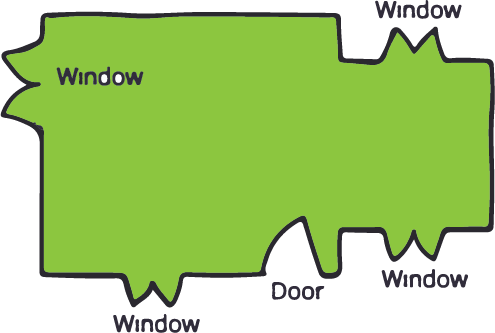Floor Maps

• The floor map is a two-dimensional picture of a home map.

• First, a floor plan of the house is created.

• It depicts the location of the house's windows and doors. The front side is the one with the door. On the front side, there are two windows.

• We can't tell how big a house is or how tall the windows are from the floor plan.

## Deep Drawings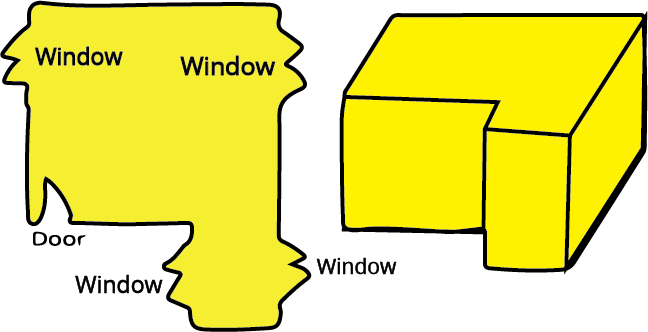Deep Drawings

• A deep drawing of a home is a specific style of sketching the house that is deep to illustrate its length, breadth, and height.

• A deep drawing of a home is a 3D depiction of a house map.

## Deep Drawing of a Cube and Cuboid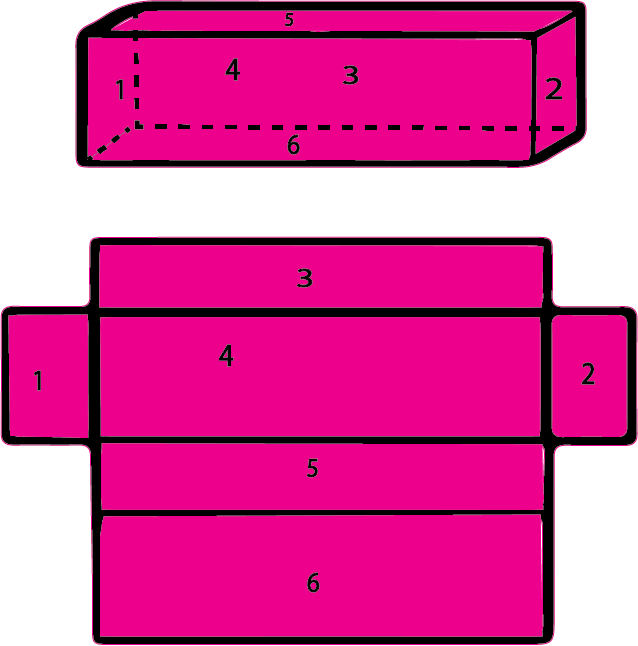Deep Drawing of a Cuboid

• Cuboid: A cuboid is a three-dimensional (3D) object with six faces. A cuboid has variable lengths, widths, and heights. A cuboid has eight corners and twelve edges.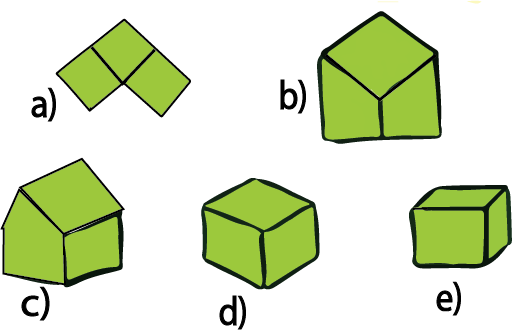Deep Drawing of a Cube

• Cube:  It is a 3D shape with 6 faces, 12 edges and 8 vertices. The above drawing of a cube shows shapes that fold into a cube.

## Visualization of 3-Dimensional Shapes

• A three-dimensional item or shape can appear differently from multiple locations (or sides), allowing them to be drawn from various viewpoints; this is known as visualizing a solid shape.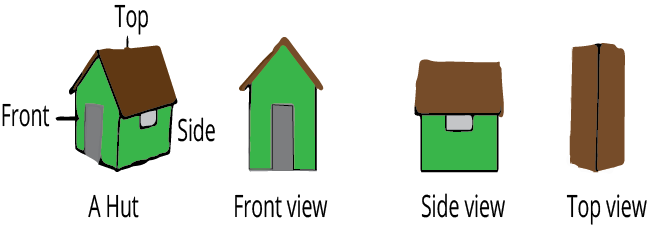Visualization of 3-Dimensional Shapes

• The front view of a hut, as illustrated above, is a combination of a square with a conical top, while the side and top views are a combination of two rectangular surfaces.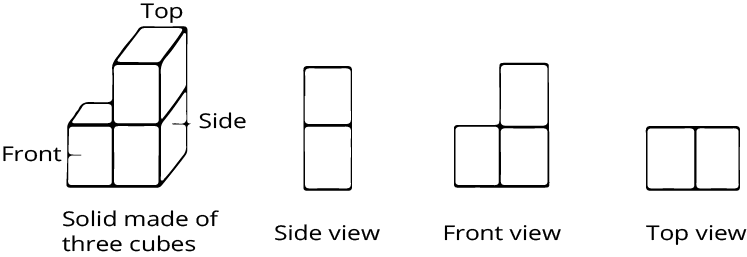Visualization of 3-Dimensional Shapes

• In the image above, one side of a solid has two square surfaces that are vertically connected, the front view has three square surfaces that are arranged in an L shape, and the top view has two square surfaces that are horizontally connected.

 Objects From Top From Side From Front House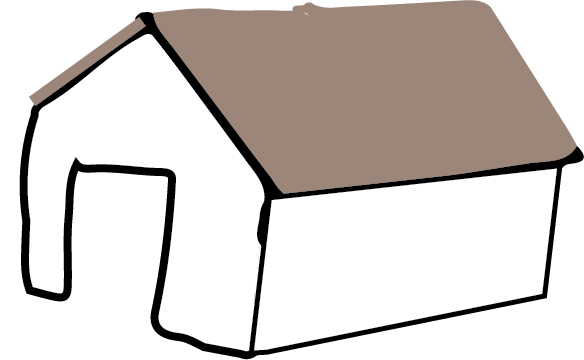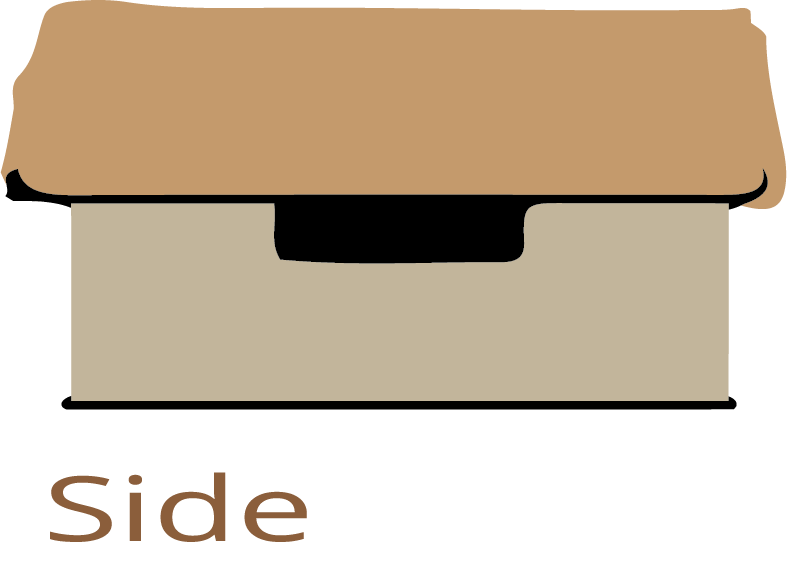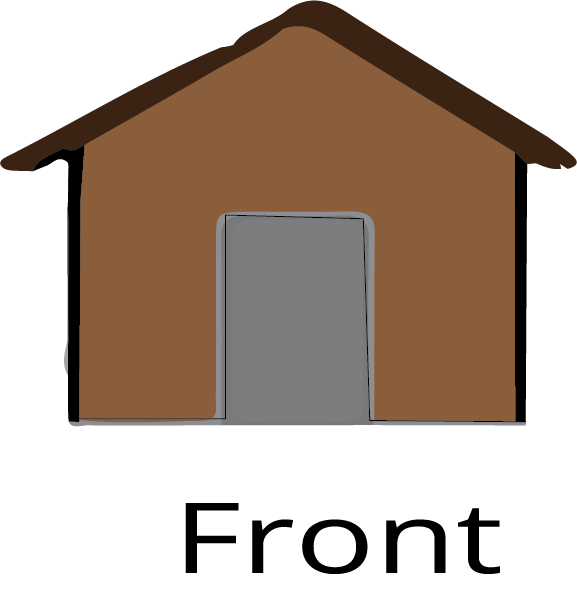) Table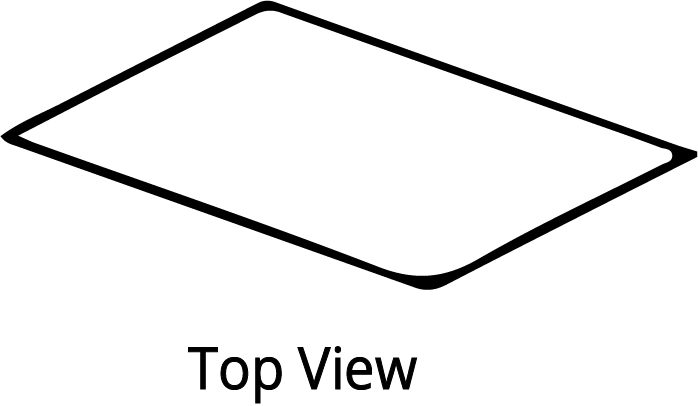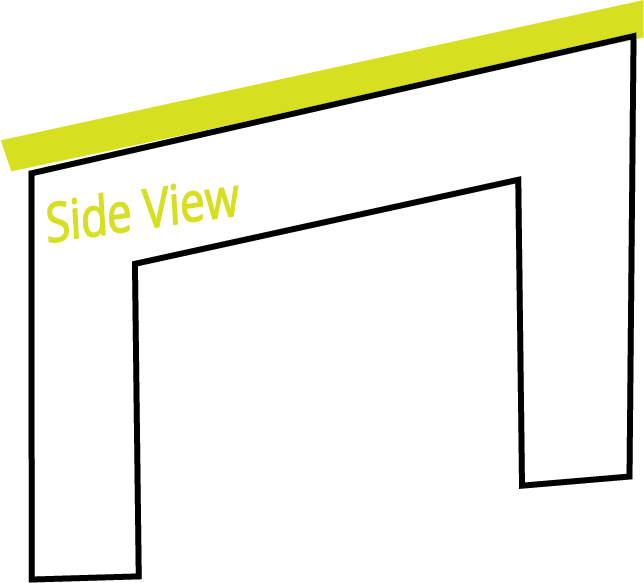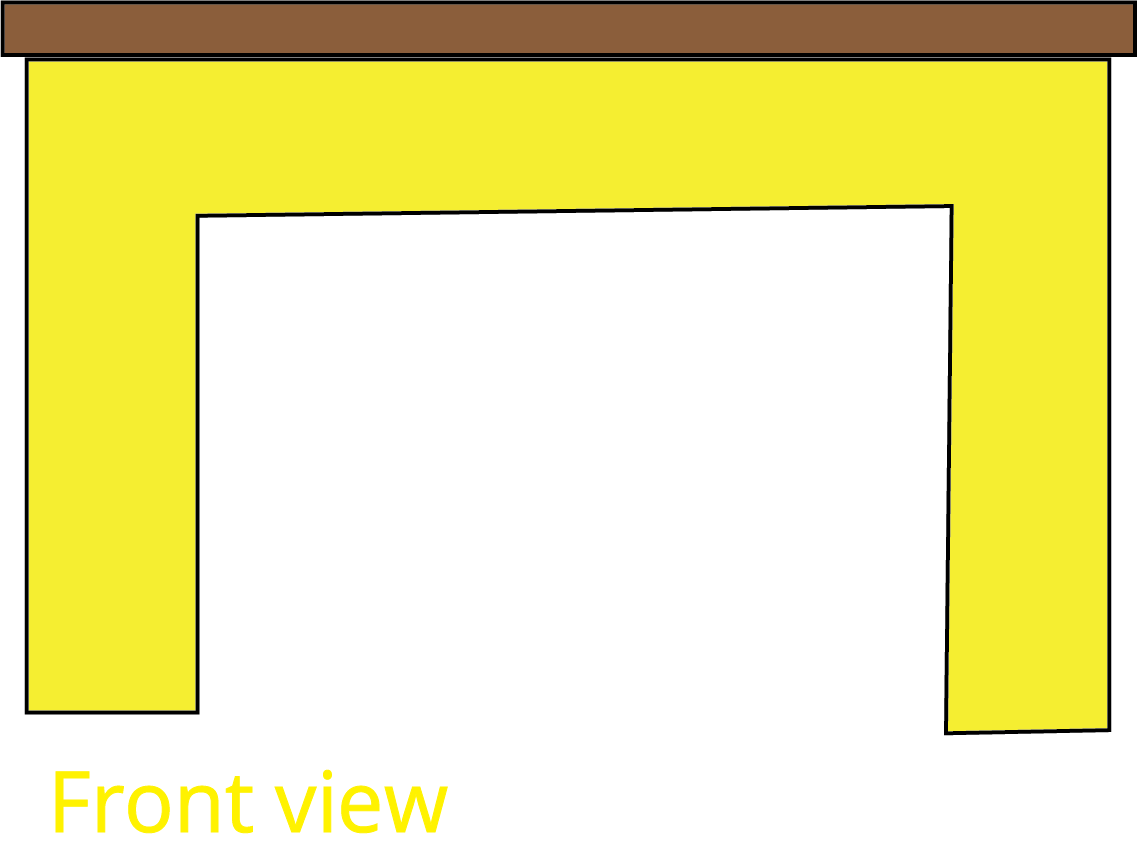## Practice Problems

1. How many sides are there in a cube?

1. 2

2. 4

3. 6

4. 8

Ans: C) 6

2. How many sides does a circle have?

1. 0

2. 1

3. 2

4. 4

Ans: A) 0

3. What is the 3D shape that can be obtained from a square?

1. Cuboid

2. Cube

3. Square-Pyramid

4. Circle

Ans: B) Cube

## Importance of CBSE Class 5 Maths Chapter 9 Boxes and Sketches

• This chapter introduces the concept of making boxes. Students will get ideas of how 3D symmetrical objects can be made following sketches on sheets and other materials.

• They will learn how to design shapes that fold into a cube. This new concept will teach students how to make a 3D object from a 2D sheet.

• Proceeding to the next part, they will understand how to create floor maps by using the given information. They will also learn to match the floor plans with the available house shapes.

• The next section will teach how a cube appears from particular angles. Students will learn how a dice looks when its surfaces are elaborated on a 2D surface. After drawing a cube, you will find out the differences between cubes and cuboids.

• Learn to draw cubes and rectangular boxes with the use of squares and rectangles. Understand the difference between cubes and boxes.

## Benefits of Boxes and Sketches Class 5 Worksheets and Revision Notes

• Our highly experienced teachers have explained the concepts of this chapter in an easy-to-understand manner. With the help of these revision notes, you will learn how to draw a cube on a sheet and make it. This experiential learning phase can be achieved with the help of Boxes and Sketches Class 5 revision notes.

• These notes will help students to relate to the objectives from the Boxes and Sketches summary and focus on the fundamental concepts defined by our subject experts.

• They can download the Boxes and Sketches Class 5 PDF worksheets for free from Vedantu and practice solving questions related to cubes and cuboids.

• The explanation of cubes and cuboids will also guide you to make different shapes with boxes at home.

## Understanding Boxes and Sketches with Revision Notes and Worksheets

Download Boxes and Sketches Class 5 worksheets with notes in the PDF version from our mobile app or website. You can study the revision notes after completing this chapter for a quick recap. Also, you can use the revision notes before an exam to revise all the concepts of this chapter. Focus on solving the worksheets to practise the commonly-asked questions from this chapter, with the help of the concepts you learn in these revision notes, and prepare well for your exams.

## FAQs on Boxes and Sketches Class 5 Notes CBSE Maths Chapter 9 (Free PDF Download)

1. What is the difference between a cube and a cuboid?

A cube has square surfaces whereas a cuboid has at least 2 rectangular surfaces. It means that a cube has all equal sides whereas a cuboid has different measures of length, breadth, and height.

2. How do I draw sketches on a sheet?

Focus on how the sketches are drawn for constructing cubes. Identify the lines where folds are to be made. It will help you make cube designs successfully on the sheets without any hassle.

3. Why should I download and use NCERT Solutions for Class 5 Maths Chapter 9?

NCERT Solutions for Class 5 Maths Chapter 9 available on Vedantu covers all exercise problems, solved by our subject experts. You will find the solutions explained in a step-wise method. Thus, it will be easier to understand the problem-solving approaches formulated by our experts for effective exam preparation.